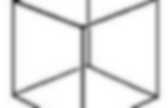# Algebraic Topology

Euler characteristic. In mathematics, and more specifically in algebraic topology and polyhedral combinatorics, the Euler characteristic (or Euler–Poincaré characteristic) is a topological invariant, a number that describes a topological space's shape or structure regardless of the way it is bent.It is commonly denoted by Polyhedra The Euler characteristic was classically defined for the surfaces of polyhedra, according to the formula This result is known as Euler's polyhedron formula or theorem. The surfaces of nonconvex polyhedra can have various Euler characteristics; This version holds both for convex polyhedra (where the densities are all 1) and the non-convex Kepler-Poinsot polyhedra. Homotopy. The two dashed paths shown above are homotopic relative to their endpoints.The animation represents one possible homotopy. Formal definition An isotopy of a coffee cup into a doughnut (torus). If we think of the second parameter of H as time then H describes a continuous deformation of f into g: at time 0 we have the function f and at time 1 we have the function g. We can also think of the second parameter as a "slider control" that allows us to smoothly transition from f to g as the slider moves from 0 to 1, and vice versa. An alternative notation is to say that a homotopy between two continuous functions f, g : X → Y is a family of continuous functions ht : X → Y for t ∈ [0,1] such that h0 = f and h1 = g, and the map (x,t) ↦ ht(x) is continuous from X × [0,1] to Y.

Properties Continuous functions f and g are said to be homotopic if and only if there is a homotopy H taking f to g as described above. Homeomorphism. A continuous deformation between a coffee mug and a donut illustrating that they are homeomorphic.But there need not be a continuous deformation for two spaces to be homeomorphic — only a continuous mapping with a continuous inverse. Topology is the study of those properties of objects that do not change when homeomorphisms are applied. As Henri Poincaré famously said, mathematics is not the study of objects, but, instead, the relations (isomorphisms for instance) between them. Definition Examples A trefoil knot is homeomorphic to a circle, but not isotopic.

Notes The third requirement, that f −1 be continuous, is essential. ) defined by f(φ) = (cos(φ), sin(φ)). For some purposes, the homeomorphism group happens to be too big, but by means of the isotopy relation, one can reduce this group to the mapping class group. Homogeneous space. Formal definition Succinctly, if X is an object of the category C, then the structure of a G-space is a homomorphism: into the group of automorphisms of the object X in the category C.The pair (X, ρ) defines a homogeneous space provided ρ(G) is a transitive group of symmetries of the underlying set of X. Examples For example, if X is a topological space, then group elements are assumed to act as homeomorphisms on X. Similarly, if X is a differentiable manifold, then the group elements are diffeomorphisms. Riemannian symmetric spaces are an important class of homogeneous spaces, and include many of the examples listed below.

Concrete examples include: Isometry groups Others Geometry From the point of view of the Erlangen program, one may understand that "all points are the same", in the geometry of X. Thus, for example, Euclidean space, affine space and projective space are all in natural ways homogeneous spaces for their respective symmetry groups. Cohomology. Definition In algebraic topology, the cohomology groups for spaces can be defined as follows (see Hatcher).Given a topological space X, consider the chain complex as in the definition of singular homology (or simplicial homology). Here, the Cn are the free abelian groups generated by formal linear combinations of the singular n-simplices in X and ∂n is the nth boundary operator. Now replace each Cn by its dual space C*n−1 = Hom(Cn, G), and ∂n by its transpose to obtain the cochain complex Then the nth cohomology group with coefficients in G is defined to be Ker(δn+1)/Im(δn) and denoted by Hn(C; G). Note that the above definition can be adapted for general chain complexes, and not just the complexes used in singular homology. Given an element φ of C*n-1, it follows from the properties of the transpose that as elements of C*n.

It can be shown that this map h is surjective, and that we have a short split exact sequence History There were various precursors to cohomology. . . Homology theory. For a topological space, the homology groups are generally much easier to compute than the homotopy groups, and consequently one usually will have an easier time working with homology to aid in the classification of spaces. The original motivation for defining homology groups is the observation that shapes are distinguished by their holes. But because a hole is "not there", it is not immediately obvious how to define a hole, or how to distinguish between different kinds of holes. Homology is a rigorous mathematical method for defining and categorizing holes in a shape. As it turns out, subtle kinds of holes exist that homology cannot "see" — in which case homotopy groups may be what is needed.